Home
Hostname: page-component-59b7f5684b-b2xwp Total loading time: 0.535 Render date: 2022-10-01T06:24:56.714Z Has data issue: true Feature Flags: { "shouldUseShareProductTool": true, "shouldUseHypothesis": true, "isUnsiloEnabled": true, "useRatesEcommerce": false, "displayNetworkTab": true, "displayNetworkMapGraph": false, "useSa": true } hasContentIssue trueCompositio Mathematica

# Rigidity of free product von Neumann algebras

Published online by Cambridge University Press:  17 November 2016

## Abstract

HTML view is not available for this content. However, as you have access to this content, a full PDF is available via the ‘Save PDF’ action button.

Let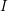$I$ be any nonempty set and let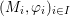$(M_{i},\unicode[STIX]{x1D711}_{i})_{i\in I}$ be any family of nonamenable factors, endowed with arbitrary faithful normal states, that belong to a large class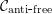${\mathcal{C}}_{\text{anti}\text{-}\text{free}}$ of (possibly type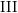$\text{III}$) von Neumann algebras including all nonprime factors, all nonfull factors and all factors possessing Cartan subalgebras. For the free product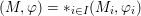$(M,\unicode[STIX]{x1D711})=\ast _{i\in I}(M_{i},\unicode[STIX]{x1D711}_{i})$, we show that the free product von Neumann algebra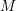$M$ retains the cardinality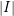$|I|$ and each nonamenable factor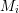$M_{i}$ up to stably inner conjugacy, after permutation of the indices. Our main theorem unifies all previous Kurosh-type rigidity results for free product type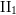$\text{II}_{1}$ factors and is new for free product type$\text{III}$ factors. It moreover provides new rigidity phenomena for type$\text{III}$ factors.

## MSC classification

Type
Research Article
Information
Compositio Mathematica , December 2016 , pp. 2461 - 2492
© The Authors 2016

## References

Anantharaman-Delaroche, C., Amenable correspondences and approximation properties for von Neumann algebras , Pacific J. Math. 171 (1995), 309341.CrossRefGoogle Scholar
Ando, H. and Haagerup, U., Ultraproducts of von Neumann algebras , J. Funct. Anal. 266 (2014), 68426913.CrossRefGoogle Scholar
Asher, J., A Kurosh-type theorem for type III factors , Proc. Amer. Math. Soc. 137 (2009), 41094116.CrossRefGoogle Scholar
Boutonnet, R., Houdayer, C. and Raum, S., Amalgamated free product type III factors with at most one Cartan subalgebra , Compos. Math. 150 (2014), 143174.CrossRefGoogle Scholar
Brown, N. P. and Ozawa, N., C -algebras and finite-dimensional approximations, Graduate Studies in Mathematics, vol. 88 (American Mathematical Society, Providence, RI, 2008).CrossRefGoogle Scholar
Chandler, B. and Magnus, W., The history of combinatorial group theory. A case study in the history of ideas, Studies in the History of Mathematics and Physical Sciences, vol. 9 (Springer, New York, 1982).CrossRefGoogle Scholar
Chifan, I. and Houdayer, C., Bass–Serre rigidity results in von Neumann algebras , Duke Math. J. 153 (2010), 2354.CrossRefGoogle Scholar
Chifan, I. and Sinclair, T., On the structural theory of II 1 factors of negatively curved groups , Ann. Sci. Éc. Norm. Supér. 46 (2013), 133.CrossRefGoogle Scholar
Connes, A., Une classification des facteurs de type III , Ann. Sci. Éc. Norm. Supér. 6 (1973), 133252.CrossRefGoogle Scholar
Connes, A., Almost periodic states and factors of type III 1 , J. Funct. Anal. 16 (1974), 415445.CrossRefGoogle Scholar
Connes, A., Classification of injective factors. Cases II 1 , II , III 𝜆 , 𝜆≠1 , Ann. of Math. (2) 74 (1976), 73115.CrossRefGoogle Scholar
Connes, A. and Jones, V. F. R., Property T for von Neumann algebras , Bull. Lond. Math. Soc. 17 (1985), 5762.CrossRefGoogle Scholar
Connes, A. and Takesaki, M., The flow of weights of factors of type III , Tôhoku Math. J. 29 (1977), 473575.CrossRefGoogle Scholar
Cowling, M. and Haagerup, U., Completely bounded multipliers of the Fourier algebra of a simple Lie group of real rank one , Invent. Math. 96 (1989), 507549.CrossRefGoogle Scholar
Gao, M. and Junge, M., Examples of prime von Neumann algebras , Int. Math. Res. Not. IMRN 2007 (2007), doi:10.1093/imrn/rnm042.Google Scholar
Ge, L., On maximal injective subalgebras of factors , Adv. Math. 118 (1996), 3470.CrossRefGoogle Scholar
Haagerup, U., Connes’ bicentralizer problem and uniqueness of the injective factor of type III 1 , Acta Math. 69 (1986), 95148.Google Scholar
Haagerup, U. and Størmer, E., Equivalence of normal states on von Neumann algebras and the flow of weights , Adv. Math. 83 (1990), 180262.CrossRefGoogle Scholar
Houdayer, C., Sur la classification de certaines algèbres de von Neumann, PhD thesis, Université Paris VII (2007).Google Scholar
Houdayer, C. and Isono, Y., Unique prime factorization and bicentralizer problem for a class of type III factors , Adv. Math. 305 (2017), 402455.CrossRefGoogle Scholar
Houdayer, C. and Raum, S., Asymptotic structure of free Araki–Woods factors , Math. Ann. 363 (2015), 237267.CrossRefGoogle Scholar
Houdayer, C. and Ueda, Y., Asymptotic structure of free product von Neumann algebras , Math. Proc. Cambridge Philos. Soc. 161 (2016), 489516.CrossRefGoogle Scholar
Houdayer, C. and Vaes, S., Type III factors with unique Cartan decomposition , J. Math. Pures Appl. 100 (2013), 564590.CrossRefGoogle Scholar
Ioana, A., Cartan subalgebras of amalgamated free product II 1 factors , Ann. Sci. Éc. Norm. Supér. 48 (2015), 71130.CrossRefGoogle Scholar
Ioana, A., Peterson, J. and Popa, S., Amalgamated free products of w-rigid factors and calculation of their symmetry groups , Acta Math. 200 (2008), 85153.CrossRefGoogle Scholar
Izumi, M., Longo, R. and Popa, S., A Galois correspondence for compact groups of automorphisms of von Neumann algebras with a generalization to Kac algebras , J. Funct. Anal. 155 (1998), 2563.CrossRefGoogle Scholar
Kadison, R. V., Diagonalizing matrices , Amer. J. Math. 106 (1984), 14511468.CrossRefGoogle Scholar
Kadison, R. V. and Ringrose, J. R., Fundamentals of the theory of operator algebras, Vol. II, Advanced theory, Graduate Studies in Mathematics, vol. 16 (American Mathematical Society, Providence, RI, 1997); pp. i–xxii and 399–1074, corrected reprint of the 1986 original.Google Scholar
Ocneanu, A., Actions of discrete amenable groups on von Neumann algebras, Lecture Notes in Mathematics, vol. 1138 (Springer, Berlin, 1985).CrossRefGoogle Scholar
Ozawa, N., Solid von Neumann algebras , Acta Math. 192 (2004), 111117.CrossRefGoogle Scholar
Ozawa, N., A Kurosh type theorem for type II 1 factors , Int. Math. Res. Not. IMRN 2006 (2006), doi:10.1155/IMRN/2006/97560.Google Scholar
Ozawa, N. and Popa, S., Some prime factorization results for type II 1 factors , Invent. Math. 156 (2004), 223234.CrossRefGoogle Scholar
Ozawa, N. and Popa, S., On a class of II 1 factors with at most one Cartan subalgebra , Ann. of Math. (2) 172 (2010), 713749.CrossRefGoogle Scholar
Peterson, J., L2 -rigidity in von Neumann algebras , Invent. Math. 175 (2009), 417433.CrossRefGoogle Scholar
Popa, S., On a problem of R.V. Kadison on maximal abelian ∗-subalgebras in factors , Invent. Math. 65 (1981), 269281.CrossRefGoogle Scholar
Popa, S., Maximal injective subalgebras in factors associated with free groups , Adv. Math. 50 (1983), 2748.CrossRefGoogle Scholar
Popa, S., Markov traces on universal Jones algebras and subfactors of finite index , Invent. Math. 111 (1993), 375405.CrossRefGoogle Scholar
Popa, S., On a class of type II 1 factors with Betti numbers invariants , Ann. of Math. (2) 163 (2006), 809899.CrossRefGoogle Scholar
Popa, S., Strong rigidity of II 1 factors arising from malleable actions of w-rigid groups I , Invent. Math. 165 (2006), 369408.CrossRefGoogle Scholar
Popa, S., On the superrigidity of malleable actions with spectral gap , J. Amer. Math. Soc. 21 (2008), 9811000.CrossRefGoogle Scholar
Popa, S. and Vaes, S., Unique Cartan decomposition for II 1 factors arising from arbitrary actions of free groups , Acta Math. 212 (2014), 141198.CrossRefGoogle Scholar
Takesaki, M., Theory of operator algebras II , in Operator algebras and non-commutative geometry, Vol. 6, Encyclopaedia of Mathematical Sciences, vol. 125 (Springer, Berlin, 2003).Google Scholar
Tomiyama, J., On some types of maximal abelian subalgebras , J. Funct. Anal. 10 (1972), 373386.CrossRefGoogle Scholar
Ueda, Y., Amalgamated free products over Cartan subalgebra , Pacific J. Math. 191 (1999), 359392.CrossRefGoogle Scholar
Ueda, Y., Remarks on free products with respect to non-tracial states , Math. Scand. 88 (2001), 111125.CrossRefGoogle Scholar
Ueda, Y., Factoriality, type classification and fullness for free product von Neumann algebras , Adv. Math. 228 (2011), 26472671.CrossRefGoogle Scholar
Ueda, Y., Some analysis on amalgamated free products of von Neumann algebras in non-tracial setting , J. Lond. Math. Soc. (2) 88 (2013), 2548.CrossRefGoogle Scholar
Vaes, S., Explicit computations of all finite index bimodules for a family of II 1 factors , Ann. Sci. Éc. Norm. Supér. 41 (2008), 743788.CrossRefGoogle Scholar
Vaes, S., Normalizers inside amalgamated free product von Neumann algebras , Publ. Res. Inst. Math. Sci. 50 (2014), 695721.CrossRefGoogle Scholar
Vaes, S. and Vergnioux, R., The boundary of universal discrete quantum groups, exactness, and factoriality , Duke Math. J. 140 (2007), 3584.CrossRefGoogle Scholar
Voiculescu, D.-V., Symmetries of some reduced free product C -algebras , in Operator algebras and their connections with topology and ergodic theory, Lecture Notes in Mathematics, vol. 1132 (Springer, 1985), 556588.CrossRefGoogle Scholar
Voiculescu, D.-V., Dykema, K. J. and Nica, A., Free random variables, CRM Monograph Series, vol. 1 (American Mathematical Society, Providence, RI, 1992).CrossRefGoogle ScholarYou have Access
13
Cited by

# Save article to Kindle

To save this article to your Kindle, first ensure coreplatform@cambridge.org is added to your Approved Personal Document E-mail List under your Personal Document Settings on the Manage Your Content and Devices page of your Amazon account. Then enter the ‘name’ part of your Kindle email address below. Find out more about saving to your Kindle.

Note you can select to save to either the @free.kindle.com or @kindle.com variations. ‘@free.kindle.com’ emails are free but can only be saved to your device when it is connected to wi-fi. ‘@kindle.com’ emails can be delivered even when you are not connected to wi-fi, but note that service fees apply.

Find out more about the Kindle Personal Document Service.

Rigidity of free product von Neumann algebras
Available formats
×

# Save article to Dropbox

To save this article to your Dropbox account, please select one or more formats and confirm that you agree to abide by our usage policies. If this is the first time you used this feature, you will be asked to authorise Cambridge Core to connect with your Dropbox account. Find out more about saving content to Dropbox.

Rigidity of free product von Neumann algebras
Available formats
×

# Save article to Google Drive

To save this article to your Google Drive account, please select one or more formats and confirm that you agree to abide by our usage policies. If this is the first time you used this feature, you will be asked to authorise Cambridge Core to connect with your Google Drive account. Find out more about saving content to Google Drive.

Rigidity of free product von Neumann algebras
Available formats
×
×